### IMO Shortlist 2008 problem A3

Kvaliteta:
Avg: 3,0
Težina:
Avg: 7,0
Let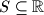$S\subseteq\mathbb{R}$ be a set of real numbers. We say that a pair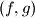$(f, g)$ of functions from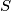$S$ into$S$ is a Spanish Couple on$S$, if they satisfy the following conditions:
(i) Both functions are strictly increasing, i.e.$f(x) < f(y)$ and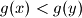$g(x) < g(y)$ for all$x$,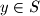$y\in S$ with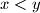$x < y$;

(ii) The inequality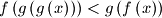$f\left(g\left(g\left(x\right)\right)\right) < g\left(f\left(x\right)\right)$ holds for all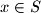$x\in S$.

Decide whether there exists a Spanish Couple on the set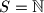$S = \mathbb{N}$ of positive integers; on the set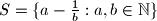$S = \{a - \frac {1}{b}: a, b\in\mathbb{N}\}$

Proposed by Hans Zantema, Netherlands
Izvor: Međunarodna matematička olimpijada, shortlist 2008